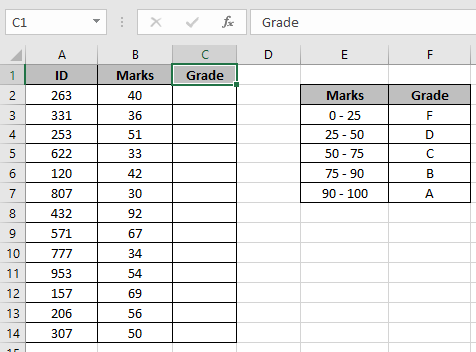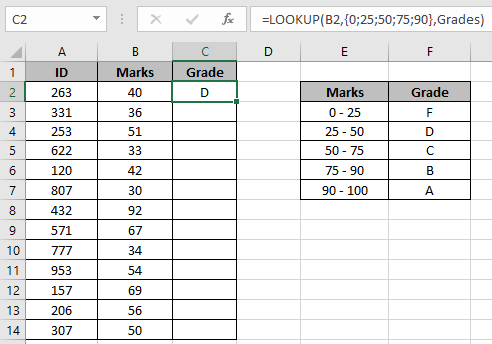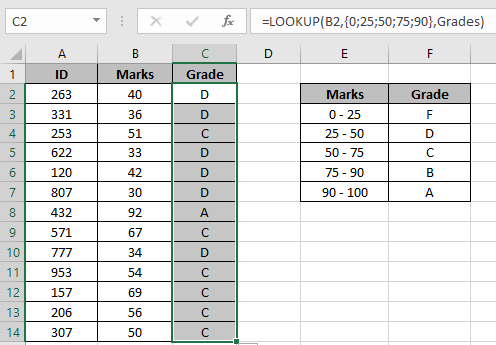# LOOKUP value between two numbers

In this article, we will learn how to LOOKUP value between two numbers in Excel.

Let's first get the knowledge about the function.

LOOKUP function finds the estimated value in the range and returns the value from the range or corresponding value.

Syntax:

=LOOKUP (lookup_value, lookup_vector, [result_vector])

lookup_value: value to look up for
lookup_vector: array where the function looks for the lookup_value.
[result vector]:[optional] if the return array is different from the lookup array.

But here we will be using its one of the attributes i.e. LOOKUP can't find a match, it will match the next smallest value. So we will be using the below syntax
Syntax:

=LOOKUP (value , {number1, number2, ...}, result_array )

This function will return values from result_array having condition between numbers.
Let’s understand this by using it in Example

Here we have a list of marks of students and we need to update the Grade column using the lookup_table.Use the formula in the C2 cell:

=LOOKUP (B2 ,{0 ; 25 ; 50 ; 75 ; 90}, Grades )

Explanation:

• If the match is found, then the function returns the value corresponding to the number (marks).
• If the match is not found, then the function finds the next large value and returns the value from just previous row.
• If the lookup value is greater than the largest value in the lookup vector, LOOKUP will return a result associated with the last value in the lookup vector.Here the Grades is named range for the F3:F7 array.

Copy the formula to the other cells using the drag down option in Excel.As you can see the updated table as the Grade column is filled with results.

Notes:

The function returns error if the argument to the function is given as cell reference.

Hope you understood how to use the LOOKUP function in Excel. Explore more articles on lookup value here. Please feel free to state your queries below in the comment box. We will certainly help you.

Related Articles

How to use LOOKUP function in Excel

How to use the VLOOKUP function in Excel

How to use the HLOOKUP function in Excel

Popular Articles

Edit a dropdown list

If with conditional formatting

If with wildcards

Vlookup by date

Terms and Conditions of use

The applications/code on this site are distributed as is and without warranties or liability. In no event shall the owner of the copyrights, or the authors of the applications/code be liable for any loss of profit, any problems or any damage resulting from the use or evaluation of the applications/code.